Next: B. Algorithms Up: A. Statistical tools Previous: A.2 Maximum likelihood

# A.3 Iterative mean

The mean value of a distribution {xi} can also be computed iteratively if the values xi are drawn one-by-one. Letx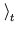be the average over the first t data points. We observe that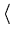x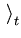=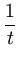xi =xi +xt =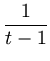xi +xt =x+xt . (A.13)

Thus, the update rule for the temporary mean c(t + 1) =xupon presentation of a value x is

 c(t + 1) = c(t) +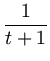x - c(t)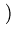. (A.14)

This rule is the same as for the center update in vector quantization with a learning rate that decays as 1/t.Next: B. Algorithms Up: A. Statistical tools Previous: A.2 Maximum likelihood
Heiko Hoffmann
2005-03-22